Homework Help Question & Answers

# i need 5 points to graph Graph()-4 log, r To graph the function, plot at least two points on the graph, draw all asymptotes, and then dick on the graph icon. Graph()-4 log, r To graph the f...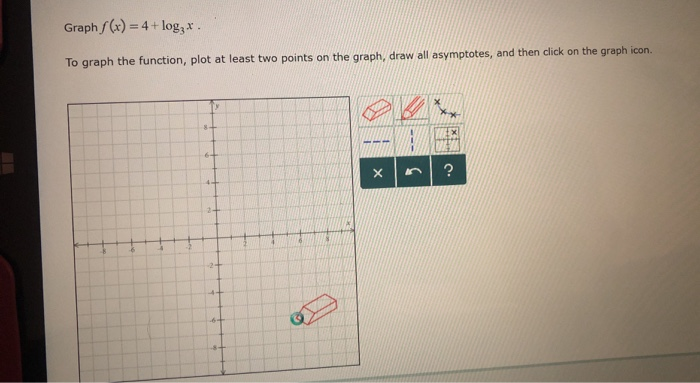i need 5 points to graph

Graph()-4 log, r To graph the function, plot at least two points on the graph, draw all asymptotes, and then dick on the graph icon.

#### Homework Answers

Answer #1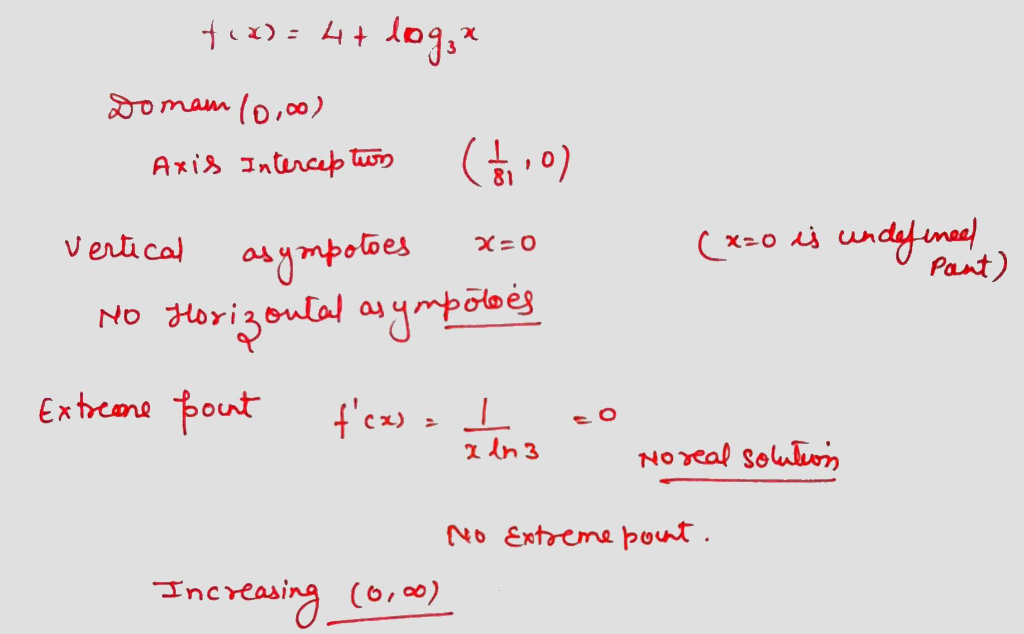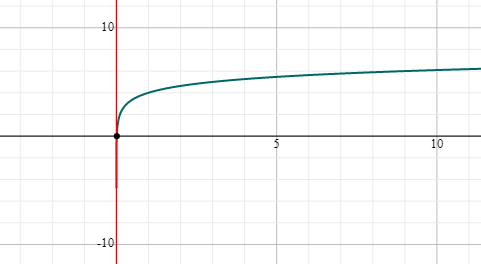Know the answer?
Your Answer:

#### Post as a guest

Your Name:

What's your source?

#### Earn Coin

Coins can be redeemed for fabulous gifts.

Not the answer you're looking for? Ask your own homework help question. Our experts will answer your question WITHIN MINUTES for Free.
Similar Homework Help Questions
• ### Graph the function. Plot all necessary asymptotes. For vertical asymptotes, make sure there are at least...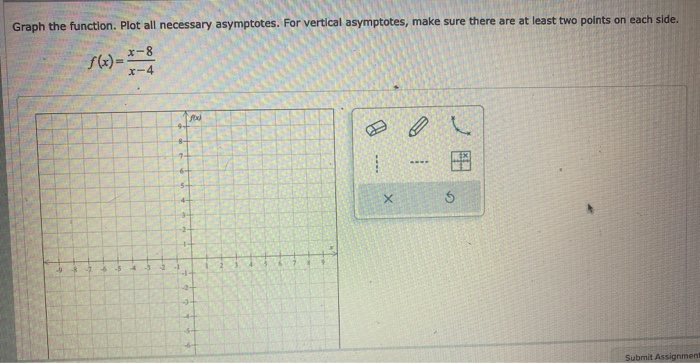Graph the function. Plot all necessary asymptotes. For vertical asymptotes, make sure there are at least two points on each side. X-8 X-4 8- 7 X 6 - Submit Assignment

• ### Graph the exponential function g(x) = ș(2)*. To graph the function, plot the points on the...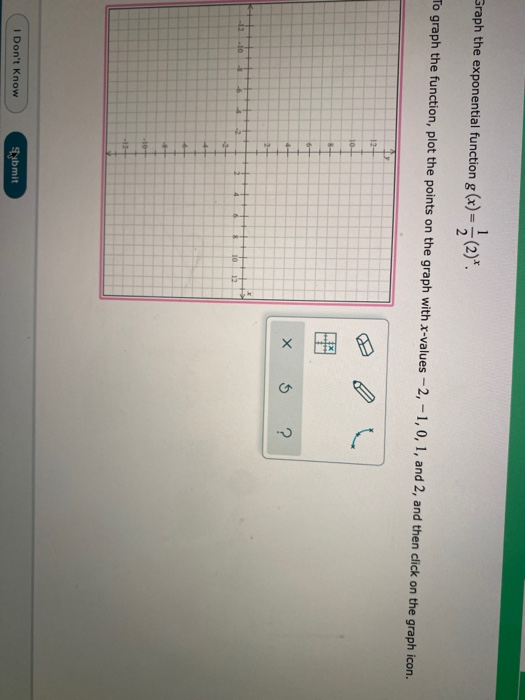Graph the exponential function g(x) = ș(2)*. To graph the function, plot the points on the graph with X-values -2, -1,0, 1, and 2, and then click on the graph icon. HER x 5 ? I Don't Know Submit

• ### 4. Find the horizontal and vertical asymptotes of the graph of the function. (You need not sketch...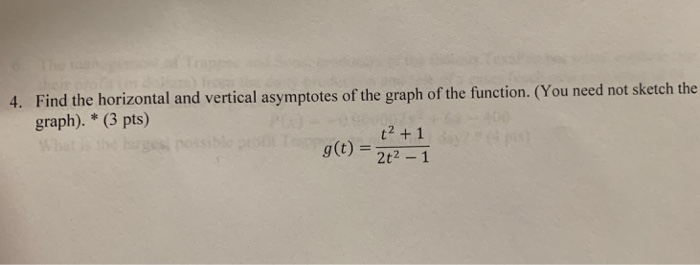help pls 4. Find the horizontal and vertical asymptotes of the graph of the function. (You need not sketch the graph). * (3 pts) g(t) = t(+1 2t2 -1 4. Find the horizontal and vertical asymptotes of the graph of the function. (You need not sketch the graph). * (3 pts) g(t) = t(+1 2t2 -1

• ### 1. (4 points) Plot a-2. Specify the coordinates used on the function graph 2. (5 points)...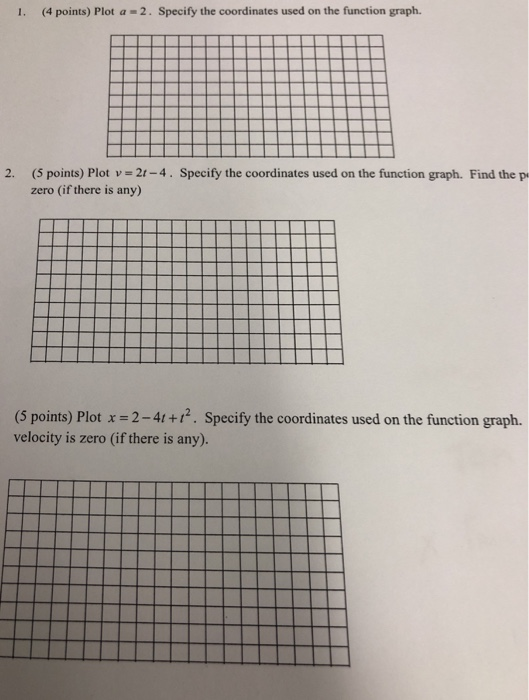1. (4 points) Plot a-2. Specify the coordinates used on the function graph 2. (5 points) Plot v- 2t-4. Specify the coordinates used on the function graph. Find the p zero (if there is any) (5 points) Plot x 2-4t+. Specify the coordinates used on the function graph. velocity is zero (if there is any).

• ### Sketch the graph of two periods of the function. Label the key points showing intercepts, asymptotes,...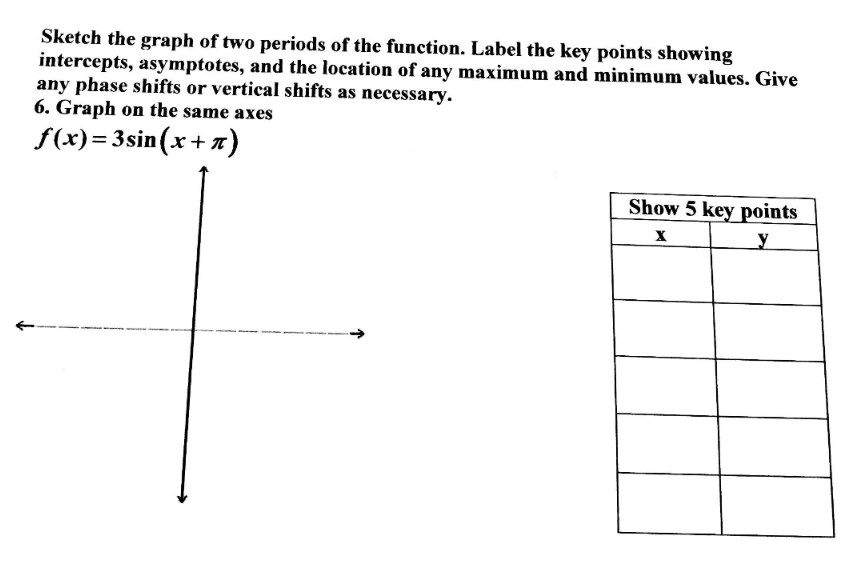Sketch the graph of two periods of the function. Label the key points showing intercepts, asymptotes, and the location of any maximum and minimum values. Give any phase shifts or vertical shifts as necessary. 6. Graph on the same axes f(x)= 3sin(x+1) Show 5 key points

• ### I need the area between the loops 4 points | Previous Answers LarCalc9 10.5.022 e a graphing utility to graph the polar equation. r-8(1 + 2 sin(θ)) 2아 15 WebAssign Plot 10 10 O 10 5 4 points...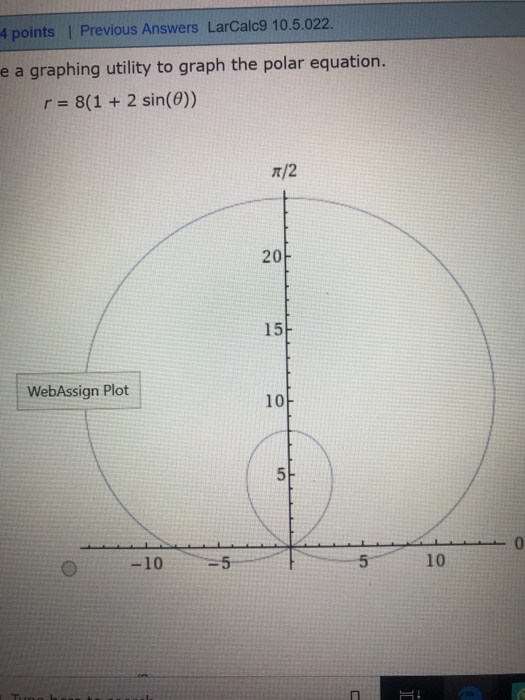I need the area between the loops 4 points | Previous Answers LarCalc9 10.5.022 e a graphing utility to graph the polar equation. r-8(1 + 2 sin(θ)) 2아 15 WebAssign Plot 10 10 O 10 5 4 points | Previous Answers LarCalc9 10.5.022 e a graphing utility to graph the polar equation. r-8(1 + 2 sin(θ)) 2아 15 WebAssign Plot 10 10 O 10 5

• ### plot the bode plot for the following I. (5 pts.) Plot the Bode plot for the following function on the graph provided. Note: you need to label the H(w axes. H)-10 +101s +100 s +10 o2π 3T/4 t/2 π/4...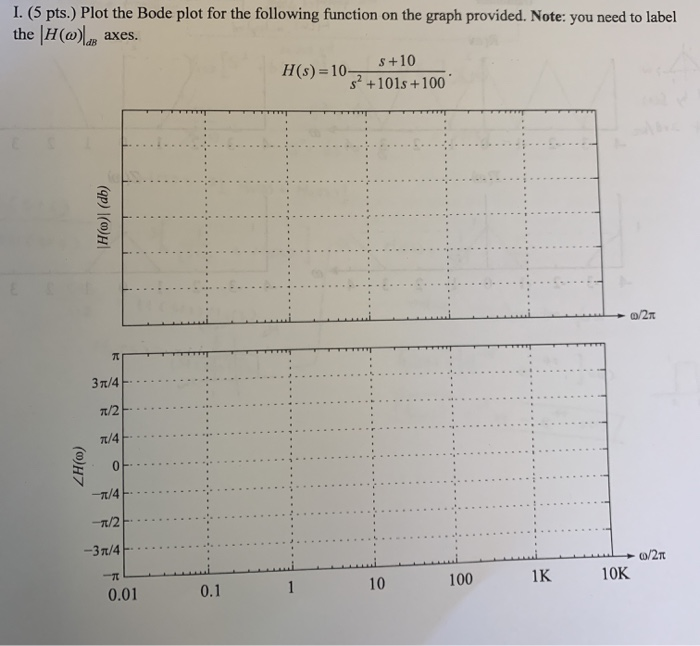plot the bode plot for the following I. (5 pts.) Plot the Bode plot for the following function on the graph provided. Note: you need to label the H(w axes. H)-10 +101s +100 s +10 o2π 3T/4 t/2 π/4 10K 1K 100 10 0.1 0.01 I. (5 pts.) Plot the Bode plot for the following function on the graph provided. Note: you need to label the H(w axes. H)-10 +101s +100 s +10 o2π 3T/4 t/2 π/4 10K 1K 100...

• ### Use a table of value and 4. Graph f(x) = log.x. plot at least 3 points....Use a table of value and 4. Graph f(x) = log.x. plot at least 3 points. у 6 5- 4- 3 2 1 3 4 5 6 X -6 -5 -4 -3 -2 -1 1 2 -3 -4 -5 1x noire -6

• ### 4. Show that a log plot is equivalent to a linear plot using the data in...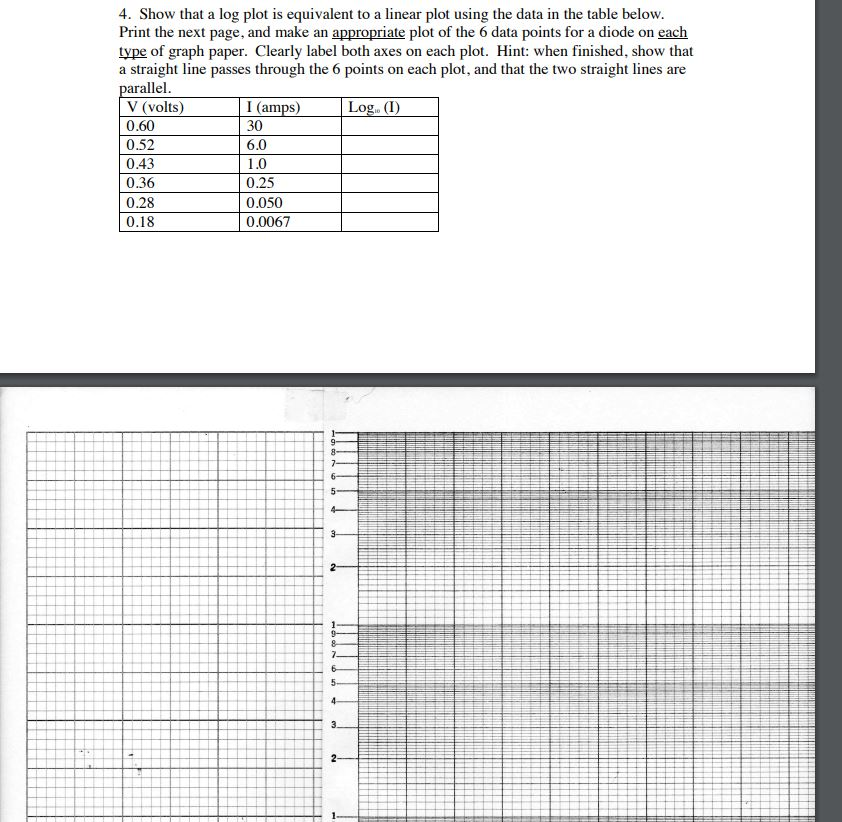4. Show that a log plot is equivalent to a linear plot using the data in the table below Print the next page, and make an appropriate plot of the 6 data points for a diode on each type of graph paper. Clearly label both axes on each plot. Hint: when finished, show that a straight line passes through the 6 points on each plot, and that the two straight lines are llel V (volts) 0.60 0.52 0.43 0.36 0.28...

• ### Find all vertical and horizontal asymptotes for the rational function f(2)= 22+1 22 Instructions: Sketch all...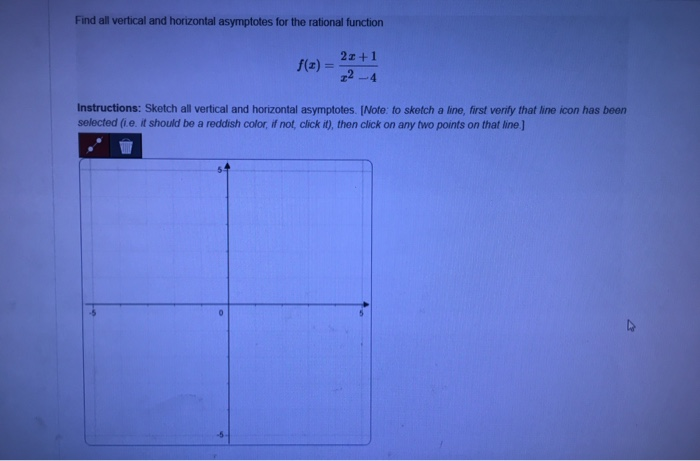Find all vertical and horizontal asymptotes for the rational function f(2)= 22+1 22 Instructions: Sketch all vertical and horizontal asymptotes. [Note: to sketch a line, first verify that line icon has been selected (ie. it should be a reddish color, if not, click it), then click on any two points on that line)

Free Homework App

Scan Your Homework
to Get Instant Free Answers
Need Online Homework Help?

Get Answers For Free
Most questions answered within 3 hours.